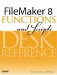# Date Calculations

#### fnAgeInYears ( dateOfBirth )

This function calculates an age in years.

The GetAsDate() function is used to ensure that whatever is passed ina date, an integer, or raw textgets converted to a date and thus accounts for data type issues.

Example:

fnAgeInYears ( "6/6/1967" ) returns 38.

Code:

``` // calculates age in years
// input: date
// output: integer
Div (Get ( CurrentDate ) - GetAsDate ( dateofbirth ) ; 365.25)
```

#### fnDateMonthEnd ( calendarDate )

This particular function determines the last day of the month for a given date. Note that in FileMaker, subtracting one from the first day of a month results in the last day of the prior month.

Example:

fnDateMonthEnd ( "1/1/2005" ) will return 1/31/2005.

Code:

``` // calculates the last day of the month for a given date
// input: date
// output: date
date ( month ( calendarDate ) + 1 ; 1; year ( calendarDate )) - 1
```

#### fnDateQuarter ( calendarDate )

This function returns numerals; if you wanted it to return text along the lines of "1st Quarter", "2nd Quarter", and so on, youd need a formula that might make use of the Choose() function.

You could also use this function to calculate fiscal quarters by adding a number at the end of the custom functions formula for whatever month begins the fiscal year for a given company.

Example:

fnDateQuarter ( 12/1/2006 ) returns 4.

Code:

``` // function returns in which calendar quarter a date falls
// input: date
// output: text

Ceiling ( Month ( calendarDate ) / 3 )

/*
```

Heres an alternative that returns text:

``` Choose ( Ceiling ( Month ( calendarDate ) / 3 ) - 1;
"1st Quarter";"2nd Quarter";"3rd Quarter";"4th Quarter" )
*/
```

#### fnDateRepetitions ( calendarDate; period; numberOfRepetitions)While wed never recommend trying to replicate the full functionality of a calendaring program like Outlook in FileMaker, we do often need to create date ranges in list form. This function will generate a delimited list of dates; you can then extract individual dates using the GetValue() function.

Note that the function requires specific keyword inputs and returns an error message if it does not recognize the value passed for its period parameter.

Example:

fnDateReptitions ( "1/1/2005"; "quarterly"; 6 ) returns

1/1/2005

4/1/2005

7/1/2005

10/1/2005

1/1/2006

4/1/2006

Code:

``` // assembles a valuelist of repeating dates based on a period keyword
// input:
// calendarDate = date
// period = "daily"; "weekly"; "monthly"; "quarterly"; "yearly"
// numberOfRepetitions = integer
// output: return-delimited list of dates as a text string

Let ([
startDate = GetAsDate ( calendarDate );
m = month (startdate);
d = day (startdate);
y = year (startdate);
nextDate = Case (
period = "daily" ; startDate + 1 ;
period = "weekly" ; startDate + 7 ;
period = "monthly" ; Date ( m + 1 ; d ; y);
period = "quarterly" ; Date ( m + 3 ; d ; y);
period = "yearly" ; Date ( m ; d ; y + 1)
)

];

Case ( numberofrepetitions > 0 ;
startdate & "¶" &
fndaterepetitions (nextdate ; period ; numberofrepetitions - 1);
"error - period not recognized"
)
)
```

#### fnNextDayOfWeek ( calendarDate; numDayOfWeek )

This function returns a future next date based on a day of week provided. For example, from a starting date of 11/2/2005, the next Friday is 11/4/2005. We often need this sort of function for reporting based on a "standard" week (week starting Friday, week starting Saturday, and the like).

The second parameter is an integer that corresponds to a day of the week. 1 = Sunday, 2 = Monday, and so on through to 7 = Saturday.

Examples:

fnNextDayOfWeek ("6/28/2006"; 7) returns the Saturday following 6/28/2006 = 7/1/2006.

fnNextDayOfWeek ("4/1/2006"; 2) returns the Monday following April Fools Day = 4/3/2006.

Code:

``` // returns the date of the next day of week requested
// input:
// calendarDate = date
// dayOfWeek = integer, 1 for Sunday ... 7 for Saturday

Let ([
varDate = GetAsDate ( calendarDate )
];
varDate + Mod ( numDayOfWeek - DayOfWeek ( varDate ); 7 )
)
```FileMaker 8 Functions and Scripts Desk Reference
ISBN: 0789735113
EAN: 2147483647
Year: 2004
Pages: 352